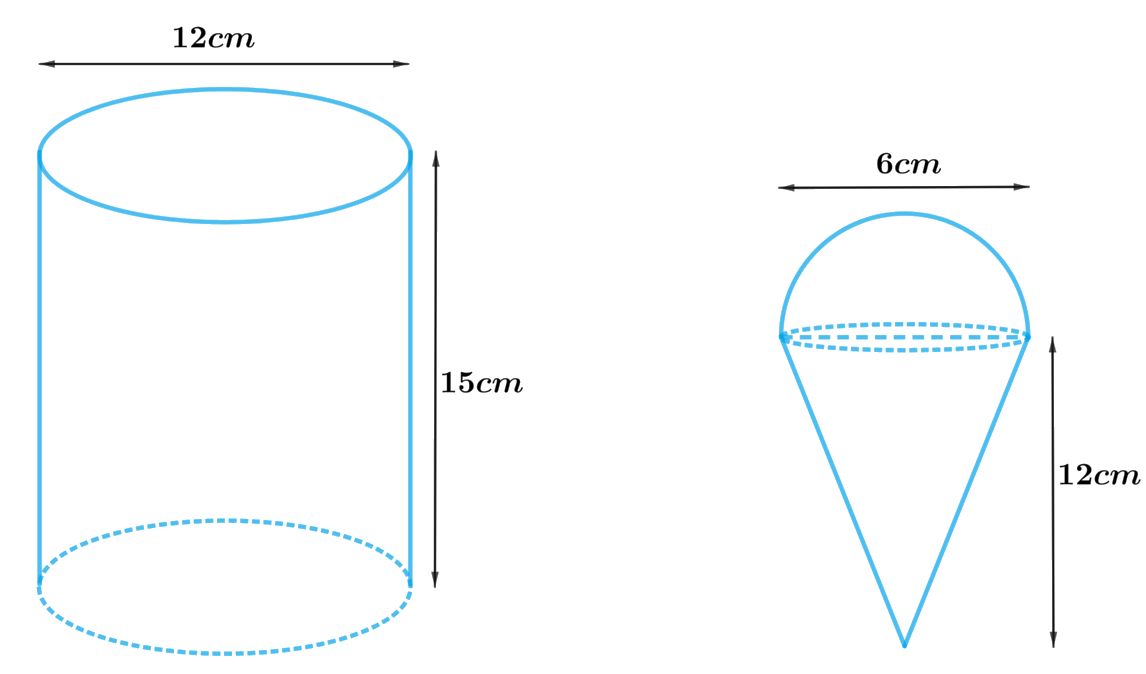# Ex.13.3 Q5 Surface Areas and Volumes Solution - NCERT Maths Class 10

Go back to  'Ex.13.3'

## Question

A container shaped like a right circular cylinder having diameter $$12\,\rm{cm}$$ and height $$15\,\rm{cm}$$ is full of ice cream. The ice cream is to be filled into cones of height $$12\,\rm{cm}$$ and diameter $$6\,\rm{cm}$$, having a hemispherical shape on the top. Find the number of such cones which can be filled with ice cream.

Video Solution
Surface Areas And Volumes
Ex 13.3 | Question 5

## Text Solution

What is known?

Diameter of the right circular cylinder is $$12\rm{cm}$$ and height is $$15\rm{cm.}$$

Diameter of the cone is $$6\rm{cm}$$ and height is $$12\rm{cm.}$$

What is unknown?

The number of cones which can be filled with ice cream.

Reasoning:

Draw a figure to visualize the shapes betterFrom the figure it’s clear that diameter of the hemisphere is same as the cone.

Since, the ice cream is to be filled into cones having a hemispherical shape on the top the volume of ice cream filled into the cone includes volume of the cone and volume of the hemisphere.

volume of the ice cream in each cone $$=$$ volume of the cone $$+$$ volume of the hemisphere

Since, the ice cream is to be filled from a cylindrical container, the volume of the ice cream filled into cones will be same as the volume of the ice cream in cylindrical container

volume of the ice cream in cylindrical container $$=$$ number of cones filled with ice cream $$\times$$ volume of the ice cream in each cone

Hence, number of cones filled with ice cream $$=$$ volume of the ice cream in cylindrical container $$\div$$volume of the ice cream in each cone

We will find the volume of the ice cream by using formulae;

Volume of the hemisphere\begin{align} = \frac{2}{3}\pi {r^3}\end{align}

where $$r$$ is the radius of the hemisphere

Volume of the cone\begin{align} = \frac{1}{3}\pi {r^2}h\end{align}

where $$r$$ and $$h$$ are the radius and height of the cone respectively

Volume of the cylinder$$= \pi {r^2}h$$

where $$r$$ and $$h$$ are radius and height of the cylinder respectively.

Steps:

Height of cylindrical container,$$H = 15 \rm m$$

Radius of cylindrical container,\begin{align} R = \frac{{12 \rm m}}{2} = 6 \rm m\end{align}

Radius of the ice-cream cone $$=$$ Radius of the hemispherical top\begin{align} = r = \frac{{6 \rm m}}{2} = 3 \rm m\end{align}

Height of the ice-cream cone,$$h = 12 \rm m$$

Let $$n$$ ice-cream cones be filled with ice-cream of the container.

volume of the ice cream in container $$= n \times$$volume of the ice cream in each cone

\begin{align}\pi {R^2}H &= n \times \begin{pmatrix} \frac{1}{3}\pi {r^2}h \\ + \frac{2}{3}\pi {r^3} \end{pmatrix}\\ \pi {R^2}H &= n\pi \left( {\frac{1}{3}{r^2}h + \frac{2}{3}{r^3}} \right)\\{R^2}H &= \frac{1}{3}n{r^2}\left( {h + 2r} \right)\\n &= \frac{{3{R^2}H}}{{{r^2}\left( {h + 2r} \right)}}\\\, &= \frac{{ \begin{bmatrix} 3 \times {{\left( {6 \rm cm} \right)}^2} \\ \times 15 \rm cm \end{bmatrix} }}{{ \begin{bmatrix}\left( 3 \rm cm \right)^2 \times \\ \begin{pmatrix} 12 \rm cm +2 \\ \times 3 \rm cm \end{pmatrix} \end{bmatrix} }}\\&= \frac{{3 \times 36 \rm c{m^2} \times 15 \rm cm}}{{9 \rm c{m^2} \times 18 cm}}\\&= 10\end{align}

Therefore, $$10$$ ice-cream cones can be filled with the ice-cream in the container.

Learn from the best math teachers and top your exams

• Live one on one classroom and doubt clearing
• Practice worksheets in and after class for conceptual clarity
• Personalized curriculum to keep up with school